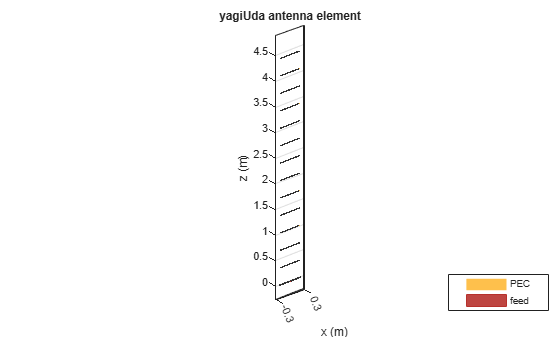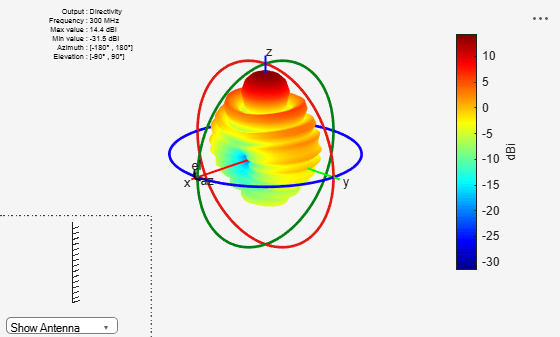# yagiUda

Create Yagi-Uda array antenna

## Description

The `yagiUda` class creates a classic Yagi-Uda array comprised of an exciter, reflector, and N- directors along the z-axis. The reflector and directors create a traveling wave structure that results in a directional radiation pattern.

The exciter, reflector, and directors have equal widths and are related to the diameter of an equivalent cylindrical structure by the equation

`$w=2d=4r$`

where:

• d is the diameter of equivalent cylinder

• r is the radius of equivalent cylinder

For a given cylinder radius, use the `cylinder2strip` utility function to calculate the equivalent width. A typical Yagi-Uda antenna array uses folded dipole as an exciter, due to its high impedance. The Yagi-Uda is center-fed and the feed point coincides with the origin. In place of a folded dipole, you can also use a planar dipole as an exciter.## Creation

### Syntax

``yu = yagiUda``
``yu = yagiUda(Name,Value)``

### Description

``` `yu = yagiUda` creates a half-wavelength Yagi-Uda array antenna along the Z-axis. The default Yagi-Uda uses folded dipole as three directors, one reflector, and a folded dipole as an exciter. By default, the dimensions are chosen for an operating frequency of 300 MHz.```

example

``` `yu = yagiUda(Name,Value)` creates a half-wavelength Yagi-Uda array antenna, with additional properties specified by one or more name-value pair arguments. `Name` is the property name and `Value` is the corresponding value. You can specify several name-value pair arguments in any order as `Name1`, `Value1`, `...`, `NameN`, `ValueN`. Properties not specified retain default values.```

## Properties

expand all

Antenna Type used as exciter, specified as the comma-separated pair consisting of `'Exciter'` and an object.

Example: `'Exciter',dipole`

Total number of director elements, specified as a scalar.

Note

Number of director elements should be less than or equal to 20.

Example: `'NumDirectors',13`

Data Types: `double`

Director length, specified as a scalar or vector in meters.

Example: `'DirectorLength',[0.4 0.5]`

Data Types: `double`

Spacing between directors, specified as a scalar or vector in meters.

Example: `'DirectorSpacing',[0.4 0.5]`

Data Types: `double`

Reflector length, specified as a scalar in meters.

Example: `'ReflectorLength',0.3`

Data Types: `double`

Spacing between exciter and reflector, specified as a scalar in meters.

Example: `'ReflectorSpacing', 0.4`

Data Types: `double`

Type of the metal used as a conductor, specified as a metal material object. You can choose any metal from the `MetalCatalog` or specify a metal of your choice. For more information, see `metal`. For more information on metal conductor meshing, see Meshing.

Example: ```m = metal('Copper'); 'Conductor',m```

Example: ```m = metal('Copper'); ant.Conductor = m```

Lumped elements added to the antenna feed, specified as a lumped element object. For more information, see `lumpedElement`.

Example: `'Load',lumpedelement`. `lumpedelement` is the object for the load created using `lumpedElement`.

Example: ```yu.Load = lumpedElement('Impedance',75)```

Tilt angle of the antenna in degrees, specified as a scalar or vector. For more information, see Rotate Antennas and Arrays.

Example: `90`

Example: `Tilt=[90 90]`,`TiltAxis=[0 1 0;0 1 1]` tilts the antenna at 90 degrees about the two axes defined by the vectors.

Data Types: `double`

Tilt axis of the antenna, specified as one of these values:

• Three-element vector of Cartesian coordinates in meters. In this case, each coordinate in the vector starts at the origin and lies along the specified points on the x-, y-, and z-axes.

• Two points in space, specified as a 2-by-3 matrix corresponding to two three-element vectors of Cartesian coordinates. In this case, the antenna rotates around the line joining the two points.

• `"x"`, `"y"`, or `"z"` to describe a rotation about the x-, y-, or z-axis, respectively.

Example: `[0 1 0]`

Example: `[0 0 0;0 1 0]`

Example: `"Z"`

Data Types: `double` | `string`

## Object Functions

 `show` Display antenna, array structures, shapes, or platform `info` Display information about antenna or array `axialRatio` Axial ratio of antenna `beamwidth` Beamwidth of antenna `charge` Charge distribution on antenna or array surface `current` Current distribution on antenna or array surface `design` Design prototype antenna or arrays for resonance around specified frequency or create AI-based antenna from antenna catalog objects `efficiency` Radiation efficiency of antenna `EHfields` Electric and magnetic fields of antennas or embedded electric and magnetic fields of antenna element in arrays `impedance` Input impedance of antenna or scan impedance of array `mesh` Mesh properties of metal, dielectric antenna, or array structure `meshconfig` Change meshing mode of antenna, array, custom antenna, custom array, or custom geometry `optimize` Optimize antenna or array using SADEA optimizer `pattern` Plot radiation pattern and phase of antenna or array or embedded pattern of antenna element in array `patternAzimuth` Azimuth plane radiation pattern of antenna or array `patternElevation` Elevation plane radiation pattern of antenna or array `rcs` Calculate and plot radar cross section (RCS) of platform, antenna, or array `returnLoss` Return loss of antenna or scan return loss of array `sparameters` Calculate S-parameters for antennas and antenna arrays `vswr` Voltage standing wave ratio (VSWR) of antenna or array element

## Examples

collapse all

Create and view a Yagi-Uda array antenna with 13 directors.

```y = yagiUda('NumDirectors',13); show(y)```Plot the radiation pattern of a Yagi-Uda array antenna at a frequency of 300 MHz.

```y = yagiUda('NumDirectors',13); pattern(y,300e6)```Calculate the width of the strip approximation to a cylinder of radius 20 mm.

`w = cylinder2strip(20e-3)`
```w = 0.0800 ```

 Balanis, C.A. Antenna Theory. Analysis and Design, 3rd Ed. New York: Wiley, 2005.